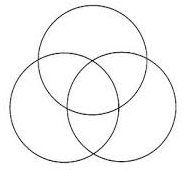# Specifying A Set

Some people will have you believe that definitions don’t really matter and we can call anything anything, but the truth is that proper definitions are empowering.

If so, can we clarify what is a set and what is meant by “specifying” it?

According to Georg Cantor a set is a collection of definite, distinguishable and distinct objects (no duplicates).

These objects are often called “elements”. More informally, they can be referred to as “things”.

So a set is a collection, or a boundary if you will, and our task is often to decide who is in, and who is out. I.e. which objects belong to the set and which don’t. This decision making is also known as “specifying” or “defining” a set. Another way of thinking of it is that we’re specifying the common characteristic that all elements of a given set have in common.

Another important thing to note is that when we talk about specifying a set, we’re really talking about a mathematical notation that enables us to identify characteristics of relevant elements. There are three main methods of mathematical notation that will allow us to do just that:

1. Enumeration – a simple list of values. Just the list and nothing but the list.
2. A calculational formula – anything that we can calculate. E.g. x-1, x/y, etc.
3. A predicate – a statement that has a truth value. E.g. x<0

These mathematical elements are combined to give us set definition notations as follows.

Notation:

The symbol ∈ is used to indicate that an element belongs to or is a member of a set. If x is an element of a set A, we write x ∈ A.

The symbol ∉ is the opposite of ∈. It is used to indicate an element that doesn’t belong to or isn’t a member of a set. If z is not an element of set A, we write z ∉ A.

Example:

If A = {1, 3, 5} then 1 ∈ A and 2 ∉ A

There are a four ways to define sets:

1. an enumerative method:
• simply a list of all the members of the set, usually within curly brackets. Sometimes this is called:

Example: {1, 3, 4, 6, 8}

• just like the first method, but uses a shorthand device “…”.

Example:

{1, 2, 3, …, 66} represents whole numbers from 1 to 66.

Or

{2, 4, 6, 8, …} represents the set of all even positive whole numbers.

1. describing the commonality of elements. This is called the set-builder notation(or the predictive method).

We describe a certain property which all the elements x of a set C have in common so that we can know whether a particular thing belongs to that set.

In this notation we introduce a concept of the “left side” and “right side”. Two sides are separated by a colon (:) or a vertical bar (|):

Left side should indicate a variable and its domain (i.e. the set that it’s chosen from).

For example – let N be a set of integers, we can define a new set S as:

S := {x ∈ N | x >0}

This is read as S is a set of elements x from a set of integers where x is greater than 0. In other words – positive integers.

Sometimes we’ll see just a variable on the left side, but really a domain is always implied.

Right side is a predicate. We can also use it to specify a domain for the variable on the left side. Our previous example would look like this:

S := {x | x ∈ N x >0}

More examples:

C := {x | x is an integer, x > – 3 }

Or

C := {x : x is an integer, x > – 3 }

This is read as: “C is a set of elements x where x is an integer greater than –3.”

D := {x | x is a street  in a city}

This is read as: “D is a set of elements x where x is a street in a city”

1. substitutive method:

Example:

A:={ x  – 1 |x N}

This should be read as follows: “the set A consists of all elements that can be calculated by a formula x -1 where x is an element of N”. In general, the left side contains an expression. The right side after the vertical bar tells you from which set you must choose the values for x to substitute in that expression.

1. a hybrid method is a combination of a set-builder notation and substitution:

Example: A:={x +1 | xS P(x) }

It can be thought of as a shorthand notation combining two steps. In this case it would be:

S1 := {xS | P(x)}  – predicative

A :={x+1| x S1} – substitutive

A simplified hybrid method can look like a predicative method. Consider, for example:

A :={x  | xS P(x) }

Looks exactly like one of the predicative example, doesn’t it?